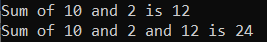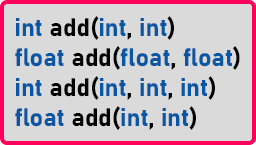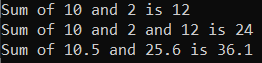# User-Defined Functions in C#

## User-Defined Functions in C# with Examples

In this article, I am going to discuss the User-Defined Functions in C# Language with Examples. Please read our previous articles discussing the Functions in C# Language with Examples. There are four types of user-defined functions in C#. They are as follows:

1. Functions with No Argument and No Return Type.
2. Functions with Argument and No Return Type.
3. Functions with No Argument and with Return Type.
4. Functions with Argument and with Return Type

Let us understand each of these function types with examples.

##### No Arguments Passed and No Return Value Function in C#:

When a function has no arguments, it does not receive any data from the calling function. Similarly, when it does not return a value, the calling function does not receive any data from the called function. So, there is no data transfer between the calling and called functions. A function that does not return any value cannot be used in an expression. It can only be used as an independent statement.

##### Example to Understand No Arguments Passed and No Return Value Function in C# Language:

In the below example, the Sum() function does not take any parameters, or even it does not return a value. The return type of the function is void. Hence, no value is returned from the function.

```using System;
namespace FunctionDemo
{
class Program
{
static void Main(string[] args)
{
Sum();
}
static void Sum()
{
int x = 10;
int y = 20;
int sum = x + y;
Console.WriteLine(\$"Sum of {x} and {y} is {sum}");
}
}
}
```

Output: Sum of 10 and 20 is 30

##### No Arguments Passed but Return a Value Function in C#:

When a function has no arguments, it receives no data from the calling function but returns a value. The calling function receives the data from the called function. So, there is no data transfer between the calling function to called function but data transfer from the called function to the calling function. The called function is executed line by line in a normal fashion until the return statement is encountered.

##### Example to Understand No Arguments Passed but Return a Value Function in C# Language:

In the below example, the empty parentheses in int Result = Sum(); statement indicates that no argument is passed to the function. And the value returned from the function is assigned to the Result variable. Here, the Sum() function will add the two numbers and returns the result.

```using System;
namespace FunctionDemo
{
class Program
{
static void Main(string[] args)
{
int Result=Sum();
Console.WriteLine(\$"Sum is {Result}");
}
static int Sum()
{
int x = 10;
int y = 20;
int sum = x + y;
return sum;
}
}
}
```

Output: Sum is 30

##### Argument Passed but no Return Value Function in C# Language:

When a function has arguments, it receives data from the calling function but does not return any value. So, there is data transfer between the calling function to called function, but there is no data transfer from the called function to the calling function. The nature of data communication between the calling function and the called function with arguments but no return value.

##### Example to Understand Argument Passed but no Return Value Function in C# Language:

In the example below, we pass two values to the Sum function, but the Sum function does not return any value to the main function.

```using System;
namespace FunctionDemo
{
class Program
{
static void Main(string[] args)
{
int x = 10, y = 20;
Sum(x, y);
}
static void Sum(int x, int y)
{
int sum = x + y;
Console.WriteLine(\$"Sum is {sum}");
}
}
}
```

Output: Sum is 30

##### Argument Passed and Return Value Function in C# Language:

A self-contained and independent function should behave like a “black box” that receives an input and outputs a value. Such functions will have two-way data communication.

##### Example to Understand Argument Passed and Return Value Function in C# Language:
```using System;
namespace FunctionDemo
{
class Program
{
static void Main(string[] args)
{
int x = 10, y = 20;
int Result = Sum(x, y);
Console.WriteLine(\$"Sum is {Result}");
}
static int Sum(int x, int y)
{
int sum = x + y;
return sum;
}
}
}
```

Output: Sum is 30

In C#, we can write more than one function with the same name, but with a different argument or parameter list, and when we do so, it is called function overloading. Let us understand this with an example.
static void main(){
int a = 10, b = 2, c;
}
This is our main function. Inside this function, we have declared 3 variables. Next, we store the result of the ‘add()’ function in the ‘c’ variable. The following is the add function.
static int add(int x, int y){
return x + y;
}
Here we haven’t declared any variable; return ‘x + y’. When we call the ‘add’ function inside the main function, then a will be copied in ‘x’, and ‘b’ will be copied in ‘y’, and it will add these two numbers, and the result will store in ‘c’. Now we want to write one more function here,
static int add(int x, int y, int z){
return x + y + z;
}
We have changed the main function as follows.
static void main(){
int a = 10, b = 2, c, d;
d = add (a, b, c);
}
Here we have created another function with the same name, ‘add’, but it takes 3 parameters. Inside the main function, we have called ‘add(x,y,z)’ and stored the result in the ‘d’ variable. So, we can have two functions with the same name but with different parameters.

So when we call “add(a, b)” it will be calling add(int x, int y), and when we call ‘add(a, b, c)’ it will be add(int x, int y, int z)”. The C# compiler can differentiate between these two functions, and this is the concept of function overloading in C#.

```using System;
namespace FunctionDemo
{
class Program
{
static void Main(string[] args)
{
int a = 10, b = 2, c, d;
Console.WriteLine(\$"Sum of {a} and {b} is {c}");
Console.WriteLine(\$"Sum of {a} and {b} and {c} is {d}");
}
static int add(int x, int y)
{
return x + y;
}
static int add(int x, int y, int z)
{
return x + y + z;
}

}
}
```
###### Output:The benefit here is that we don’t have to think of new names every time. As both functions are for adding integers, so we don’t have to give different names. It is easy to write the programs, and you don’t have to remember too many function names. That is the benefit we are getting now. Let us declare one more add function, which returns the sum of two float numbers.

static float add(float x, float y){
return x + y;
}

This function will return the float type value. Now we have two add functions that are taking the same number of parameters. Is it possible in C#? Yes, two functions can have the same name and the same number of parameters, but the data type of the parameters should be different. They cannot be the same.

So int add (int x, int y) and float add (float x, float y) are two different functions. In C#, two functions are said to be different if they have the same name but different parameters list.

##### How Can Be the Parameters List Different?

Either the data type of the parameter or the number of parameters. For a better understanding, please have a look at the below image.Above are the signatures of the different ‘add’ functions. Now let us check which is valid or which is invalid.

1. int add(int, int) is valid; it takes 2 ‘int’ type parameters and returns the ‘int’ value.
2. float add(float, float) is valid as it takes 2 ‘float’ parameters and returns the ‘float’ value. It takes the same number of parameters but different data types as compared to the first one.
3. int add(int, int, int) is valid as it takes 3 ‘int’ parameters and returns the ‘int’ value. It takes a different number of parameters but has the same data types as compared to the first one.
4. float add(int, int) is invalid; it is the same as the first function, which takes the same number of parameters and the same type of parameters. So, this is invalid. It doesn’t matter what type of data a function is returning. If two functions have the same number of parameters and of the same type, then this is invalid.
```using System;
namespace FunctionDemo
{
class Program
{
static void Main(string[] args)
{
int a = 10, b = 2, c, d;
Console.WriteLine(\$"Sum of {a} and {b} is {c}");
Console.WriteLine(\$"Sum of {a} and {b} and {c} is {d}");
Console.WriteLine(\$"Sum of 10.5 and 25.6 is {add(10.5f, 25.6f)}");
}
static int add(int x, int y)
{
return x + y;
}
static int add(int x, int y, int z)
{
return x + y + z;
}
static float add(float x, float y)
{
return x + y;
}
}
}
```
###### Output: